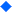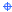# Math

###General TopicsA+ Math
Games, Flash cards and a homework helper are to be found here.
http://www.aplusmath.com/Academy Curricular Exchange: Mathematics Intermediate (6-8)
The OFCN's resource of math lesson plans.Aims Puzzles
Site is updated often.
http://www.aimsedu.org/Puzzle/PuzzleList.htmlAmerican Mathematical Society's E-Math Site
http://e-math.ams.orgBamdad's Math Comics
http://www.csun.edu/~hcmth014/comics.html?BasketMathBinary Magic
Game
http://emsh.calarts.edu/~juan/game.html GameBiographies of Women Mathematicians
http://www.agnesscott.edu/lriddle/women/women.htmBrain Binders
http://www.brainbinders.com/Brain Teasers in Math
The brain teasers are broken down by grade level and allow student to submit their answers.
http://www.eduplace.com/math/brain/index.htmlBrain Tricks
A new puzzle every week.
http://www.braintricks.com/Center for Polymer Studies
A site with Fractal activities.
http://polymer.bu.edu/Chronological List of Mathematicians
http://aleph0.clarku.edu:80/~djoyce/mathhist/chronology.html?Dave's Math Tables
Site contains a complete set of table for every math discipline.
http://www.sisweb.com/math/tables.htm?Dictionary of Units of Measurement
http://www.unc.edu/~rowlett/units/index.htmlEisenhower National Clearinghouse
http://www.enc.org/ ?Euclid's Elements, Introduction
http://aleph0.clarku.edu/~djoyce/java/elements/elements.html?Fibonacci Numbers and Nature
http://www.ee.surrey.ac.uk/Personal/R.Knott/Fibonacci/fibnat.htmlFlash Cards for Kids
http://www.edu4kids.com/?TB=1&page=11Funbrain.com
http://www.funbrain.comGo Airborne with Plane Math
http://www.planemath.com?History of Math
ttp://lcweb.loc.gov/exhibits/vatican/math.html?Hot list: Mathematics
http://sln.fi.edu/tfi/hotlists/math.htmlIntegers, Rational & Irrational Numbers
Explanation with examples.
http://forum.swarthmore.edu/dr.math/faq/faq.integers.htmlInteractive Mathematics - 36 Games and Puzzles
http://www.cut-the-knot.com/Los Alamos National Laboratory Math Site
Site contains problems, games, and unusual anecdotes to teach the rules of logic, graphing, and other mathematical concepts
http://www.c3.lanl.gov/mega-math/gloss/gloss.html?Mankala
Game site.
http://www.elf.org/Mankala.htmlMath File
Online, math-related games
http://www.bbc.co.uk/education/mathsfile/intro.htmlMath Forum: Math Tips Tricks
http://forum.swarthmore.edu/k12/mathtips/index.htmlMath Problems for Kids
http://www.stfx.ca/special/mathproblems/welcome.htmlMathMagic!
http://forum.swarthmore.edu/mathmagic/Math Forum
http://forum.swarthmore.edu?Math League
http://www.mathleague.com?Math Magic
http://forum.swarthmore.edu/mathmagicMagic Squares, Magic Stars
http://www.geocities.com/~harveyh/Mathpuzzle.com?
http://www.mathpuzzle.com/Mathematical Quotations Server
http://math.furman.edu/~mwoodard/mquot.html? ?Mathematics?
http://www.useekufind.com/learningquest/tmath.htmMathematics Lessons
http://math.rice.edu/~lanius/Lessons/Mathline from PBS
http://www.pbs.org/learn/mathline/? ?MathMol K12
Introduction to the field of molecular modeling and its application to mathematics
.http://www.nyu.edu/pages/mathmol/?Mike's Page of Math Problems
http://www.charm.net/~shack/math?New Math Teachers
Great site for new teachers.
http://people.clarityconnect.com/webpages/terri/terri.htmlNegative X Negative = Postive
http://forum.swarthmore.edu/dr.math/faq/faq.negxneg.htmlNumber Puzzles
Sort of a numbers based crossword puzzle site.
http://www1.tpgi.com.au/users/puzzles/page2.htmlOrder of Operations
Way to explain with examples
http://www.blarg.net/~math/order.htmlPavilion of Polyhedreality
3-D technology used here.
http://www.georgehart.com/Prentice Hall Mathematics: Tools For a Changing World
Teacher and student sections Teachers may use the chapter by chapter resource to locate the Internet activities relating to each concept. There are links to support materials, references, and professional development. You need not use their text in order to benefit from the site.
http://www.phschool.com/atschool/Mathematics/Algebra/Teacher_Area/ALG_T_BK_index.htmlPrime Page
http://www.utm.edu/research/primes/index.html?PI
Explanation of and activities relating to "pi".
http://www.ncsa.uiuc.edu:80/edu/RSE/RSEorange/ ?Plane Math
Combination of math and aeronautics.
http://www.planemath.com/Probability
http://www.mathgoodies.com/lessons/toc_vol6.htmlSMG2000 Stock Market Game for Kids
http://www.smg2000.org?SOS Mathematics -
Lessons and related practice problems.
http://www.sosmath.com/Square Roots without a Calculator
http://forum.swarthmore.edu/dr.math/faq/faq.sqrt.by.hand.htmlTeachers First
Classroom humor, teacher time savers and lesson plans.
http://www.teachersfirst.com/Texas Instrument Education Site
http://www.ti.com/calc/docs/calchome.htmlTexas Instruments Calculators
http://www.ticalc.org?The 100th Day
Site dealing with the concept of 100.
http://www.siec.k12.in.us/~west/proj/100th/index.htmlThe Brain and Behavior
A interactive site.
http://serendip.brynmawr.edu/bb/The Gateway to Educational Materials
Giant lesson PLAN site.
http://www.thegateway.org/The Fruit Game?
A game where you find the pattern in order to win.
http://www.2020tech.com/fruit/The History of Mathematics
http://www.maths.tcd.ie/pub/HistMath/HistMath.htmlTI Calculators and Educational Solutions
Site for teachers, students, and parents.
http://www.ti.com/calc/docs/calchome.htmlWorld of Mathematics
Encyclopedic treatment.
http://mathworld.wolfram.com/

# Middle School Math

Middle school is one of the best times to begin exploring mathematics: this subject for many middle school kids is scary. Thus, getting rid of this fear of middle school math from the students can be a tough job for the teachers. Apart from handling the students, the teacher should be well aware of the particulars they need to cover without going over and beyond the required level. Middle school math contains topics such as: fractions, geometry, formulas, conversions and general math. Mathematics at middle school level should teach students to intelligently logic out the formulas and apply math in their day-to-day life.

Teaching math at middle school level can be very challenging. Teaching concepts, which are applicable in real life, is a tough job, but with the collection of resources and questions to practice, the fear of math in students can be tackled. This guide will provide various links to learn the topics and links to various worksheets, which students can practice to judge their understanding of fundamentals and concepts on each of the topics.

Learning mathematics at any level can be fun provided proper concepts are embedded in the minds of those who teach and in those who study them. Middle school math concepts can be easy to grab at times but just knowing these concepts won’t fetch grades, worksheets are a must if you wish to come out with flying colors in the league of mathematics.

Fractions

Fractions in mathematics are the ratio of algebraic quantity expressed in a/b form. In middle school, the child should be familiar with concepts of fractions and identifying a fraction. They should be learning about equivalent and identical fractions. The basic formulas of adding, subtracting, multiplying and dividing should be imbibed. Students should also be able to compare fractions and convert them into decimals.

Word problems are the most difficult ones to tackle. Conversion between various units can be asked from pupils. The students also desire converting decimals into fractions.

Geometry

Geometry is a branch of mathematics all about shape, size, relative position of figures and the properties of space. Middle school geometry introduces topics such as: polygons, area, perimeter and volume of geometric and solid figures. Topics on angles, coordinate planes and transformation should be introduced here. Congruency and similarity of triangles are the other topics covered under this unit.

Algebra

Understanding the concepts of coefficients, variables and polynomials are expected of the students here. This section of math introduces solving of algebraic equations Evaluation of algebraic polynomials along with the application of basic mathematics formula on the expressions are needed. This is a tough section and usually students take time before they settle in this section.

Other mathematics

In mathematics, a percentage is a way of expressing a number as a fraction of 100. It is denoted by "%" symbol. This concept of math is applied in daily life as well; therefore having a grasp on this subject is essential. Students are required to calculate percentages of amount given to them. Word problems form a mandatory part of this section.

• Learning percentage - A simple interactive guide to learn percentages.
• Tutorials - This guide provides all the formula pertaining to this topic in math.
• Interactive percentage – A webpage with explanation of how to use a 10 x 10 grid to explain basic concept of percent, and solve various types of percent problems
• Math Quizzes - An interactive quiz on percentage of numbers

This unit in mathematics covers all the basic operations in the domain on integers. Integers are natural numbers including 0 with all the negatives of the non-zero natural numbers. Here, basic mathematics formulas should also be taught to the students.